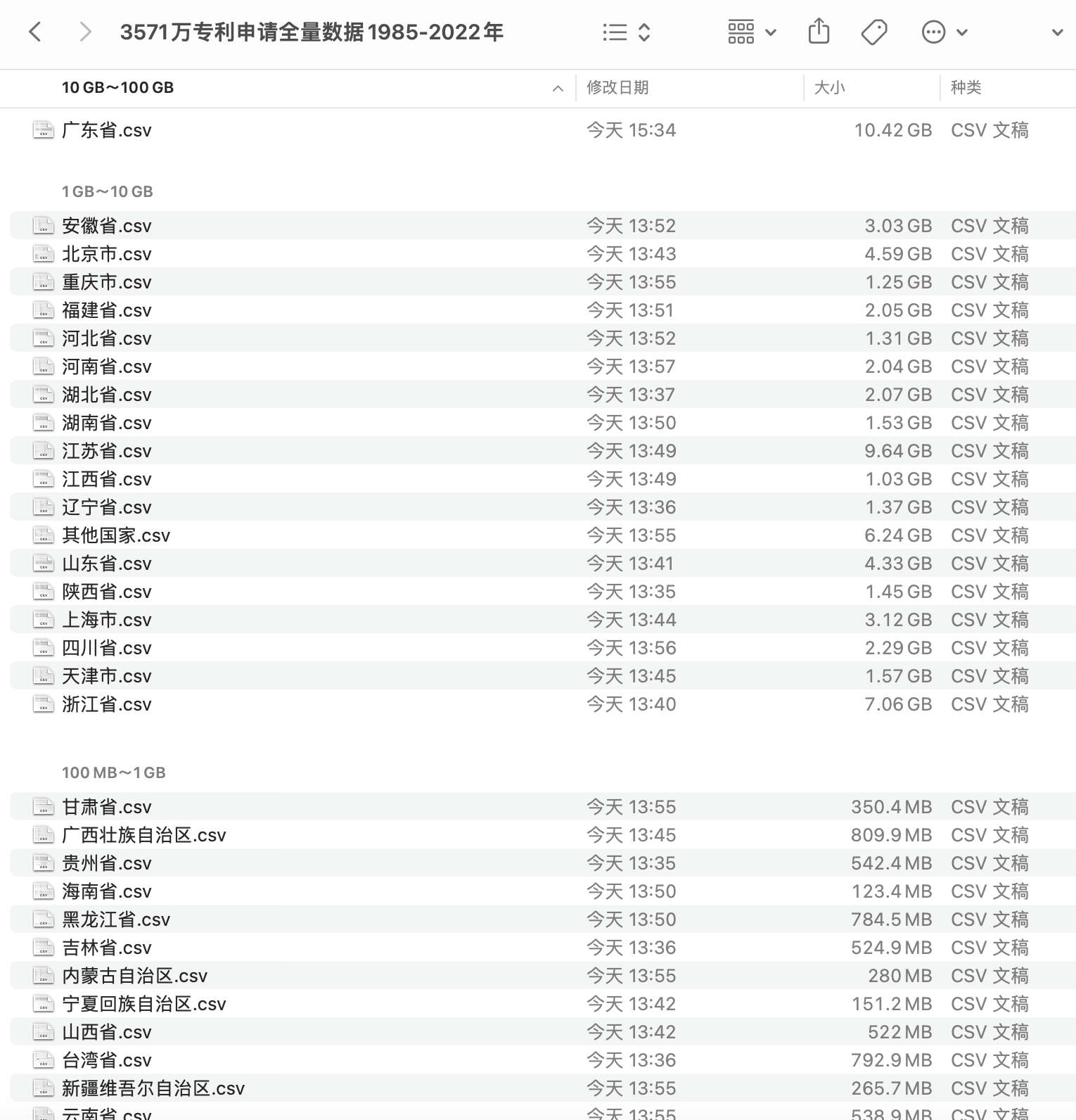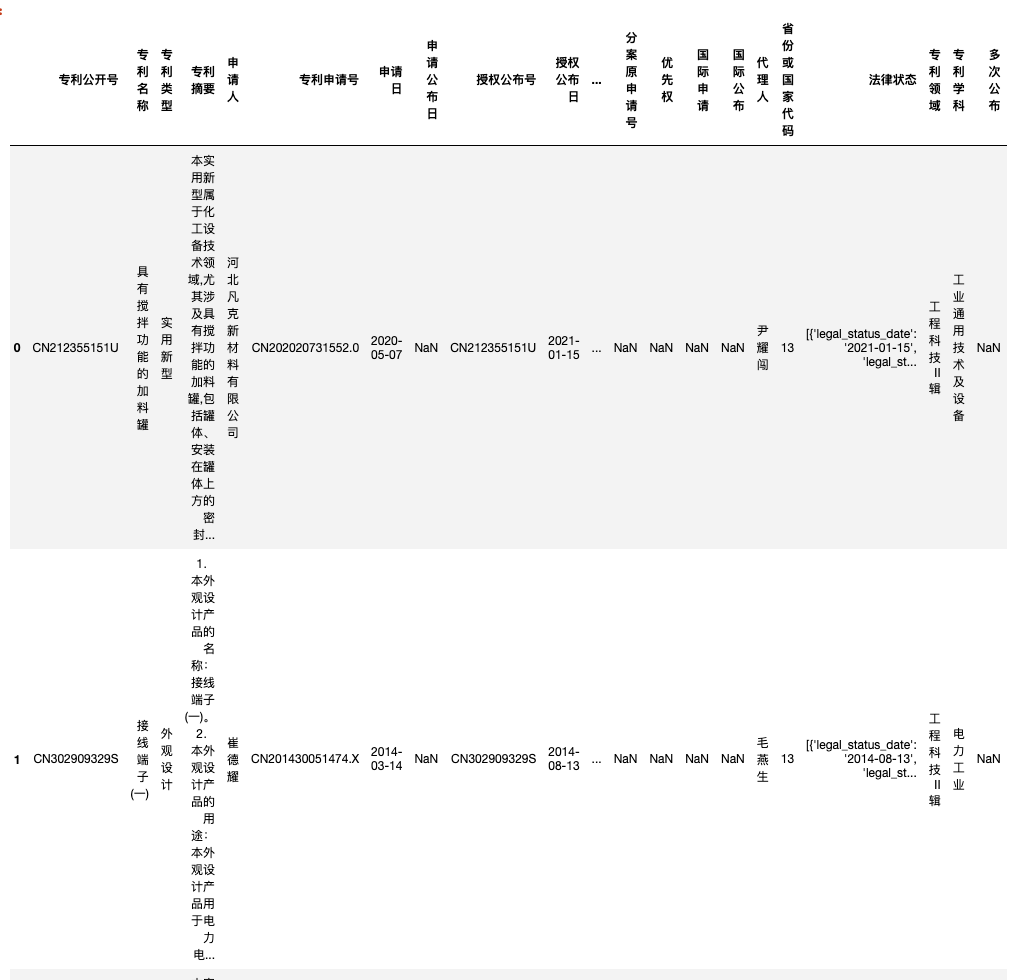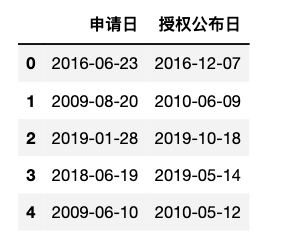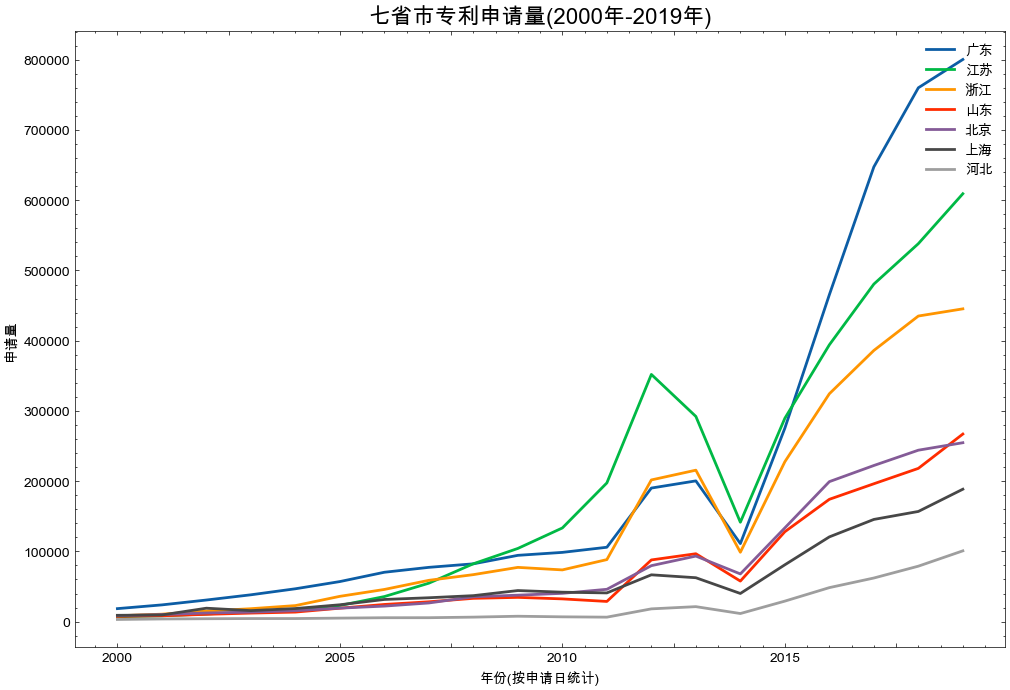## 相关推文#

3571万专利申请全量数据(1985.01 ~ 2022.5)数据，解压后整个文件夹70多G。## 一、数据介绍#

### 1.1 数据集概况#

- 数据集名称：省份版知识产权局专利
- 时间跨度：1985.1-2022.5，专利申请总量3571万
- 数据来源：『国家知识产权局』
- 数据整理: 『公众号:大邓和他的Python』


### 1.2 分省统计#

| 省份(区域)       |  专利数  |
| :---------------| :------ |
| 广东省           | 5728705 |
| 江苏省           | 4879171 |
| 浙江省           | 3706820 |
| 山东省           | 2064446 |
| 北京市           | 2069913 |
| 四川省           | 1159551 |
| 天津市           | 712932  |
| 上海市           | 1548278 |
| 贵州省           | 265512  |
| 陕西省           | 655837  |
| 吉林省           | 232264  |
| 辽宁省           | 637853  |
| 湖北省           | 966384  |
| 山西省           | 233418  |
| 宁夏回族自治区    | 66919   |
| 西藏自治区        | 9911    |
| 广西壮族自治区    | 377658  |
| 江西省           | 519584  |
| 湖南省           | 743828  |
| 黑龙江省         | 357881  |
| 海南省           | 59202   |
| 福建省           | 1046473 |
| 安徽省           | 1342364 |
| 河北省           | 645420  |
| 重庆市           | 592382  |
| 内蒙古自治区      | 133277  |
| 云南省           | 252407  |
| 甘肃省           | 164274  |
| 新疆维吾尔自治区   | 124734  |
| 河南省           | 966477  |
| 青海省           | 34127   |
| 台湾省           | 401555  |
| 香港特别行政区    | 61636   |
| 澳门特别行政区    | 2010    |
| 其他国家         | 2948557 |


### 1.3 字段#

 -  专利公开号
-  专利名称
-  专利类型
-  专利摘要
-  申请人
-  专利申请号
-  申请日
-  申请公布日
-  授权公布号
-  授权公布日
-  申请地址
-  主权项
-  发明人
-  分类号
-  主分类号
-  代理机构
-  分案原申请号
-  优先权
-  国际申请
-  国际公布
-  代理人
-  省份或国家代码
-  法律状态
-  专利领域
-  专利学科
-  多次公布


## 二、读取数据#

import pandas as pd

df['申请日'] = pd.to_datetime(df['申请日'])


Runprint('河北省: ', len(df))


Run

河北省: 645420


print(df['申请日'].min())
print(df['申请日'].max())


Run

1985-01-28 00:00:00
2022-05-24 00:00:00


df.columns


Run

Index(['专利公开号', '专利名称', '专利类型', '专利摘要',
'申请人', '专利申请号', '申请日', '申请公布日',
'授权公布号', '授权公布日', '申请地址', '主权项', '发明人',
'分类号', '主分类号', '代理机构', '分案原申请号', '优先权',
'国际申请', '国际公布', '代理人', '省份或国家代码',
'法律状态', '专利领域', '专利学科', '多次公布'],
dtype='object')


df['专利类型'].value_counts()


Run

实用新型 361832



## 三、可视化#

import pandas as pd

#为减轻内存压力，可以选择需要的字段读取
cols = ['申请日', '授权公布日']

#读取数据
guangdong_df = pd.read_csv('广东省.csv', usecols=cols, encoding='utf-8', low_memory=False)
jiangsu_df = pd.read_csv('江苏省.csv', usecols=cols, encoding='utf-8', low_memory=False)
shandong_df = pd.read_csv('山东省.csv', usecols=cols, encoding='utf-8', low_memory=False)
zhejiang_df = pd.read_csv('浙江省.csv', usecols=cols, encoding='utf-8', low_memory=False)
beijing_df = pd.read_csv('北京市.csv', usecols=cols, encoding='utf-8', low_memory=False)
shanghai_df = pd.read_csv('上海市.csv', usecols=cols, encoding='utf-8', low_memory=False)

#显示前5行import matplotlib.pyplot as plt
import matplotlib
import matplotlib_inline
matplotlib_inline.backend_inline.set_matplotlib_formats('png', 'svg')
import scienceplots
import platform
plt.style.use(['science', 'no-latex', 'cjk-sc-font'])
system = platform.system()  # 获取操作系统类型

if system == 'Windows':
font = {'family': 'SimHei'}
elif system == 'Darwin':
font = {'family': 'Arial Unicode MS'}
else:
font = {'family': 'sans-serif'}
matplotlib.rc('font', **font)  # 设置全局字体

plt.figure(figsize=(12, 8))
years = [str(y) for y in range(2000, 2020)]

guangdong_df['申请日'].str.slice(0, 4).value_counts(ascending=True)[years].plot(label='广东', linewidth=2)
jiangsu_df['申请日'].str.slice(0, 4).value_counts(ascending=True)[years].plot(label='江苏', linewidth=2)
zhejiang_df['申请日'].str.slice(0, 4).value_counts(ascending=True)[years].plot(label='浙江', linewidth=2)
shandong_df['申请日'].str.slice(0, 4).value_counts(ascending=True)[years].plot(label='山东', linewidth=2)
beijing_df['申请日'].str.slice(0, 4).value_counts(ascending=True)[years].plot(label='北京', linewidth=2)
shanghai_df['申请日'].str.slice(0, 4).value_counts(ascending=True)[years].plot(label='上海', linewidth=2)
hebei_df['申请日'].str.slice(0, 4).value_counts(ascending=True)[years].plot(label='河北', linewidth=2)

plt.title('七省市专利申请量(2000年-2019年)', fontsize=16, color='black', ha='center')
plt.xlabel('年份(按申请日统计)')
plt.ylabel('申请量')
plt.legend(loc='upper right')
plt.show()• 2012年， 申请量开始下降， 直至2014年，触底反弹。这个时期国内外宏观经济发生了什么？

• 不考虑人口规模， 在专利申请量可以看出广东、江苏、浙江体量还是比北京、上海、河北要高的。

• 2015年开始， 广东触底反弹后， 拉开了与江苏、浙江的体量。